# How to Add an EditField Component in MATLAB?

MATLAB allows us to create graphical user interface (GUI) applications without proper knowledge of coding. It has a built-in component library containing a wide range of GUI components such as buttons, edit fields, numeric edit fields, hyperlinks, and many more. In this tutorial, I will explain how you can create an EditField component in MATLAB.

## What is an EditField Component in MATLAB?

In MATLAB, the EditField component is a graphical user interface (GUI) component which is used to allow your users to input and edit text or number. The EditField component is one the fundamental GUI components used to build GUI apps in MATLAB.

### Methods of Creating an EditField Component in MATLAB

We can use any of the following two methods:

• MATLAB App Designer

• 'uieditfield()' Function

Let us now discuss all these methods of creating Edit Field component in MATLAB along with examples.

## Create an EditField Component using MATLAB App Designer

The MATLAB App Designer is a built-in environment in MATLAB which provides a GUI interface for creating GUI applications just through drag and drop of components.

Here is the step-by-step process for creating an EditField using the App Designer in MATLAB:

Step (1) – Open MATLAB and launch the App Designer.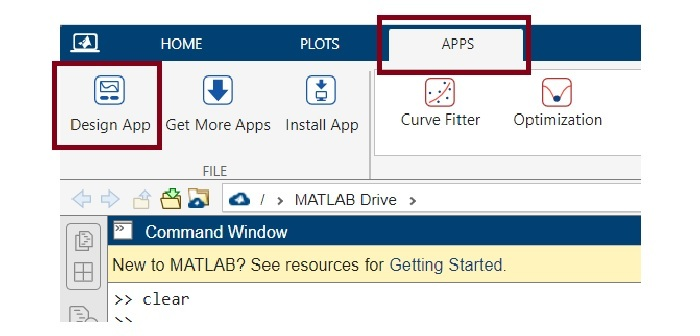Step (2) – Create a blank app.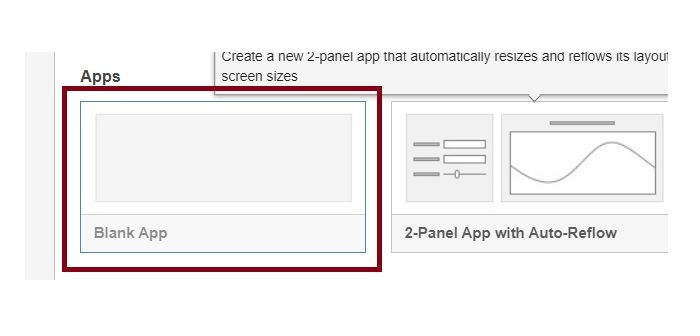Step (3) – Once you have created a black app, your computer screen will look like this.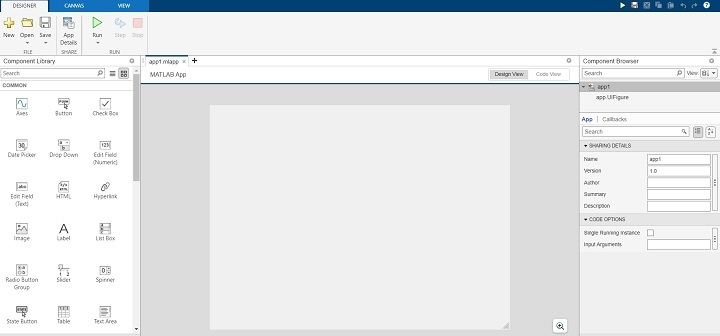Step (4) – Now, you can drag and drop the EditField component from the component library. You can pick either Numeric EditField or Text EditField based on requirements.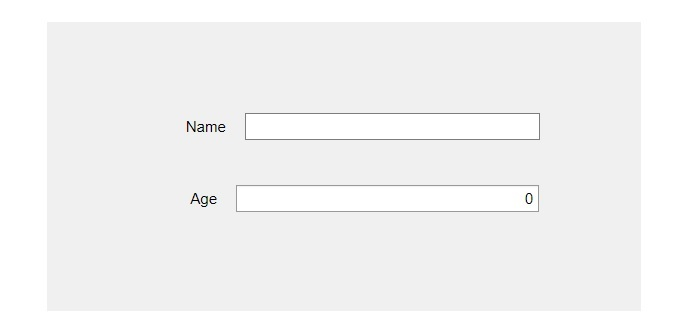Step (5) – In this step, resize the component and place it at the desired location on the canvas.

Step (6) - Customize the EditField component by changing its properties given in the right-side pane.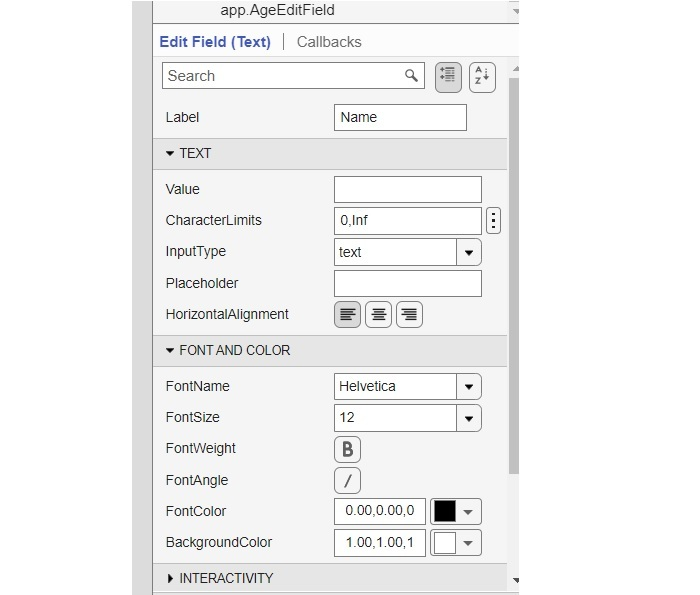### MATLAB Code

The MATLAB code for the above EditField component that we have created in the above steps is given below:

classdef app1 < matlab.apps.AppBase

% Properties that correspond to app components
properties (Access = public)
UIFigure            matlab.ui.Figure
NameEditField       matlab.ui.control.EditField
NameEditFieldLabel  matlab.ui.control.Label
AgeEditField        matlab.ui.control.NumericEditField
AgeEditFieldLabel   matlab.ui.control.Label
end

% Component initialization
methods (Access = private)

% Create UIFigure and components
function createComponents(app)

% Create UIFigure and hide until all components are created
app.UIFigure = uifigure('Visible', 'off');
app.UIFigure.Position = [100 100 640 480];
app.UIFigure.Name = 'MATLAB App';

% Create AgeEditFieldLabel
app.AgeEditFieldLabel = uilabel(app.UIFigure);
app.AgeEditFieldLabel.HorizontalAlignment = 'right';
app.AgeEditFieldLabel.Position = [155 230 26 22];
app.AgeEditFieldLabel.Text = 'Age';

% Create AgeEditField
app.AgeEditField = uieditfield(app.UIFigure, 'numeric');
app.AgeEditField.Position = [196 230 243 22];

% Create NameEditFieldLabel
app.NameEditFieldLabel = uilabel(app.UIFigure);
app.NameEditFieldLabel.HorizontalAlignment = 'right';
app.NameEditFieldLabel.Position = [151 288 37 22];
app.NameEditFieldLabel.Text = 'Name';

% Create NameEditField
app.NameEditField = uieditfield(app.UIFigure, 'text');
app.NameEditField.Position = [203 288 236 22];

% Show the figure after all components are created
app.UIFigure.Visible = 'on';
end
end

% App creation and deletion
methods (Access = public)

% Construct app
function app = app1

% Create UIFigure and components
createComponents(app)

% Register the app with App Designer
registerApp(app, app.UIFigure)

if nargout == 0
clear app
end
end

% Code that executes before app deletion
function delete(app)

% Delete UIFigure when app is deleted
delete(app.UIFigure)
end
end
end


This is how we can easily add an EditField component (Text or Numeric) in our MATLAB application using the App Designer tool.

Now, let us discuss another method of creating an EditField using the 'uieditfield' function.

## Create an EditField Component using 'uieditfield' Function

In MATLAB, there is a built-in function 'uieditfield' which allows us to create an EditField component. This function has different syntaxes depending on the use case. Commonly use syntaxes of the 'uieditfield' function are given below:

• e = uieditfield

• e = uieditfield(style)

• e = uieditfield(parent)

• e = uieditfield(parent,style)

• e = uieditfield(___,Name,Value)

Let us discuss about each of these syntaxes in detail.

## Create an EditField Component with Default Properties

We use the following syntax of the 'uieditfield' function to create an EditField component with default properties.

e = uieditfield();


### Example

Consider the following example to understand the implementation of this syntax.

% MATLAB code to create an EditField with default properties
e = uieditfield();


### Output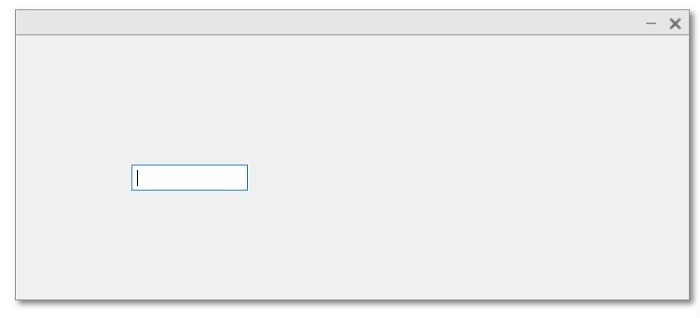## Create an EditField Component with Specified Style

Create an EditField Component with Specified Style:

e = uieditfield(style);


### Example

The following example illustrates the implementation of this syntax:

% MATLAB program to create an EditField component with specified style
% Create a numeric edit field
en = uieditfield('numeric');


### Output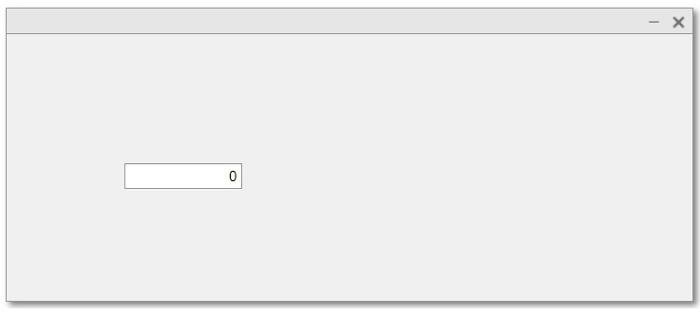## Create an EditField Component within a Specified Parent Container

The following syntax of the 'uieditfield' function is used to create an EditField component within a specified parent container.

e = uieditfield(parent);


### Example

The following MATLAB program describes the code implementation for this function:

% Create a figure as parent container
fig = uifigure('Name', 'TutorialsPoint');

% Create the EditField contain within the parent
e = uieditfield(fig);


### Output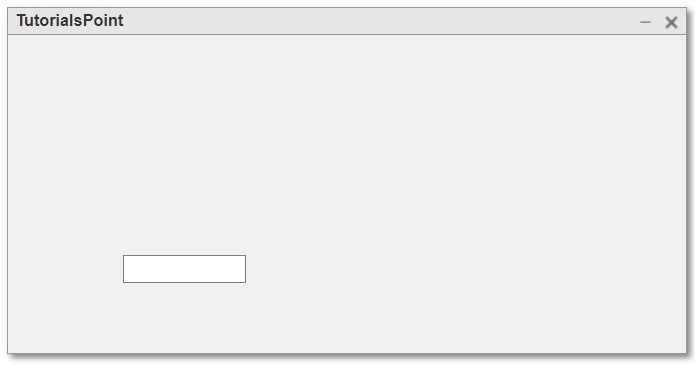## Create an EditField Component with a specified style within a Specified Parent Container

The following syntax of the 'uieditfield' function is used to create an EditField component with a specified style within a specified parent container.

e = uieditfield(parent, style);


### Example

The following MATLAB program describes the code implementation for this function:

% Create a figure as parent container
fig = uifigure('Name', 'TutorialsPoint');

% Create a numeric edit field
en = uieditfield(fig, 'numeric');


### Output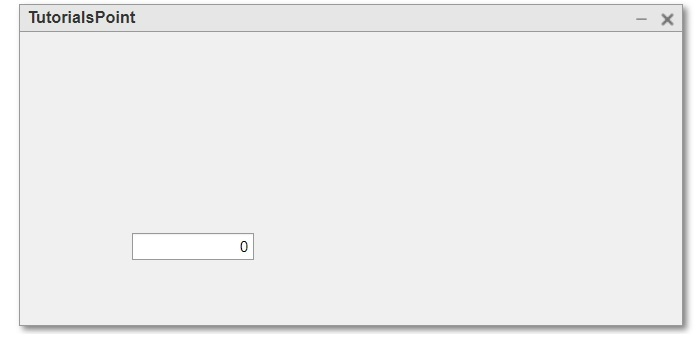## Create an EditField Component with specified properties

The following syntax of the 'uieditfield' function is used to create an EditField component with specified properties.

e = uieditfield(---, Name, Value,…);


Here, the name-value pair is used to specify the property of the EditField component.

The code implementation of this function is demonstrated in the following example.

### Example

% MATLAB program to create EditField components with specific properties
% Create a parent container
fig = uifigure('Name', 'TutorialsPoint');

% Create a numeric EditField
en = uieditfield(fig, 'numeric', 'Value', 50, 'Position', [100, 100, 150, 25]);

% Create a text EditField
et = uieditfield(fig, 'Value', 'Type your name...', 'Position', [100, 150, 150, 25]);



### Output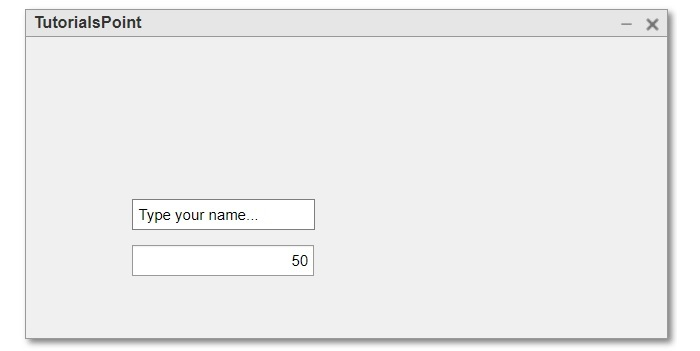## Conclusion

This is all about creating EditField component in MATLAB. In conclusion, an EditField component is a GUI component used in MATLAB applications to allow user to input and edit text and numbers. In this tutorial, I explained different methods to create an EditField component in a MATLAB application.

Updated on: 07-Sep-2023

30 Views